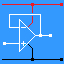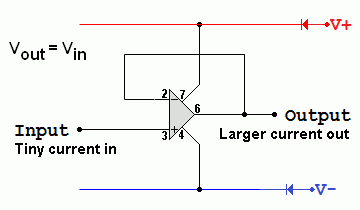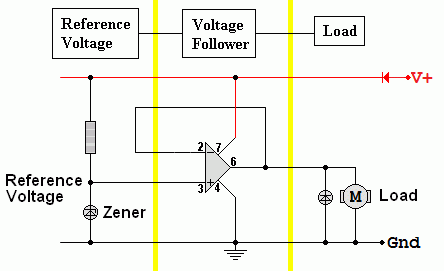RANDOM PAGE

SITE SEARCH

LOG
IN

HELP

# Voltage Follower

This is the AQA version closing after June 2019. Visit the the version for Eduqas instead.

## Voltage Follower Properties

• Voltage gain = 1.
• Input resistance = Infinity.
• Output resistance is low.
• Vout is equal to Vin.

Voltage followers are used to boost the current available from a circuit without increasing the voltage at the same time.

The circuit can be used as a buffer or driver. This isolates the output circuit so the input is not affected in any way by the output device.

If you have a circuit that is capable of providing plenty of voltage but little current, adding a voltage follower will increase the current that can be supplied. The voltage follower does not amplify the voltage but the output current can be much higher than that of the original circuit.• Vout = Vin
• Vout / Vin = 1
• The input resistance is equal to that of the op-amp. (Close to infinity).
• The output resistance is low (Close to zero). In reality it depends on the op amp and values near 200 Ohms are common. High power modules are available with much lower output resistances.

For the Falstad Circuit Simulation, CTRL+Click Voltage Follower
In options, check European Resistors and uncheck Conventional Current.

Alternatively view Voltage_Follower.txt.
Save or copy the text on the web page. Import the saved or copied text into the Falstad simulator.

Here is the new HTML5 Simulator Site.

The input current = zero. The output current is quite large. There is lots of current gain.

For the mathematically inclined, a voltage follower is a non inverting amplifier where ...

• Rf = 0 (it is a piece of wire!) This provides negative feedback.
• R1 = ∞ (Infinity, it is a piece of air (not there at all))
• Gain = 1 + Rf / R1
• Gain = 1 + 0 / ∞
• Gain = 1

## Voltage Follower Example

Here a Zener diode is providing a reference voltage such as 9 volts. The current available from this reference voltage circuit is minimal. The voltage follower outputs the same reference voltage but with enough current to supply the motor. If the motor load increases, the voltage follower maintains the same output voltage but allows more current to flow in the motor. Stabilised power supplies work like this.The Zener diode needs to have about 5 or 10 mA breakdown current flowing through it. This ensures that the Zener voltage will be correctly established.

reviseOmatic V3     Contacts, ©, Cookies, Data Protection and Disclaimers Hosted at linode.com, London